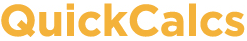1. Select category 2. Choose calculator 3. Enter data 4. View results

## Analyze a 2x2 contingency table

Enter the number of subjects actually observed. Don't enter proportions, percentages or means.

### Which test

There are three ways to compute a P value from a contingency table. Fisher's test is the best choice as it always gives the exact P value, while the chi-square test only calculates an approximate P value. Only choose chi-square if someone requires you to. The Yates' continuity correction is designed to make the chi-square approximation better. With large sample sizes, the Yates' correction makes little difference. With small sample sizes, chi-square is not accurate, with or without the correction.

Fisher's exact test (recommended)
Chi-square with Yates' correction
Chi-square without Yates' correction

A P value can be calculated with either one or two tails. We suggest always using two-tailed (also called two-sided) P values. Read more about P values .

Two-tailed (recommended)
One-tailed